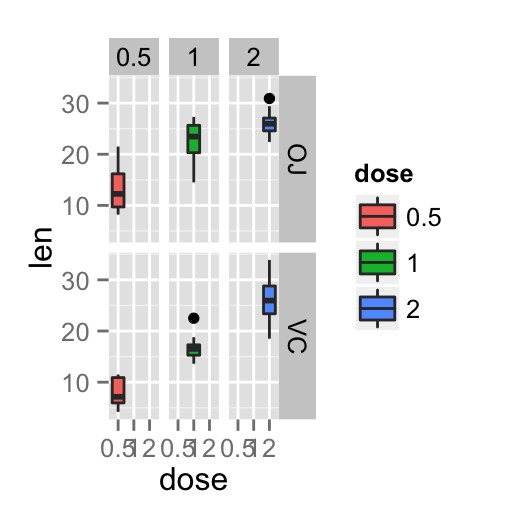# ggplot2 facet : split a plot into a matrix of panels

The facet approach partitions a plot into a matrix of panels. Each panel shows a different subset of the data. This R tutorial describes how to split a graph using ggplot2 package.

There are two main functions for faceting :

• facet_grid()
• facet_wrap()

# Data

ToothGrowth data is used in the following examples.

``````# Convert dose from numeric to factor variables
ToothGrowth\$dose <- as.factor(ToothGrowth\$dose)
df <- ToothGrowth
``````##    len supp dose
## 1  4.2   VC  0.5
## 2 11.5   VC  0.5
## 3  7.3   VC  0.5
## 4  5.8   VC  0.5
## 5  6.4   VC  0.5
## 6 10.0   VC  0.5``````

Make sure that the variable dose is converted as a factor using the above R script.

# Basic box plot

Create a basic box plot filled by groups :

``````library(ggplot2)
bp <- ggplot(df, aes(x=dose, y=len, group=dose)) +
geom_boxplot(aes(fill=dose))
bp``````# Facet with one variable

The graph is partitioned in multiple panels by levels of the group “supp”:

``````# Split in vertical direction
bp + facet_grid(supp ~ .)
# Split in horizontal direction
bp + facet_grid(. ~ supp)``````# Facet with two variables

The graph is partitioned by the levels of the groups “dose” and “supp” :

``````# Facet by two variables: dose and supp.
# Rows are dose and columns are supp
bp + facet_grid(dose ~ supp)
# Facet by two variables: reverse the order of the 2 variables
# Rows are supp and columns are dose
bp + facet_grid(supp ~ dose)``````Note that, you can use the argument margins to add additional facets which contain all the data for each of the possible values of the faceting variables

``bp + facet_grid(dose ~ supp, margins=TRUE)``# Facet scales

By default, all the panels have the same scales (`scales="fixed"`). They can be made independent, by setting scales to `free`, `free_x`, or `free_y`.

``bp + facet_grid(dose ~ supp, scales='free')``As you can see in the above plot, y axis have different scales in the different panels.

# Facet labels

The argument labeller can be used to control the labels of the panels :

``bp + facet_grid(dose ~ supp, labeller=label_both)``The appearance of facet labels can be modified as follow :

``````# Change facet text font. Possible values for the font style:
#'plain', 'italic', 'bold', 'bold.italic'.
bp + facet_grid(dose ~ supp)+
theme(strip.text.x = element_text(size=12, color="red",
face="bold.italic"),
strip.text.y = element_text(size=12, color="red",
face="bold.italic"))
# Change the apperance of the rectangle around facet label
bp + facet_grid(dose ~ supp)+
theme(strip.background = element_rect(colour="black", fill="white",
size=1.5, linetype="solid"))``````# facet_wrap

Facets can be placed side by side using the function facet_wrap() as follow :

``````bp + facet_wrap(~ dose)
bp + facet_wrap(~ dose, ncol=2)``````# Infos

This analysis has been performed using R software (ver. 3.1.2) and ggplot2 (ver. 1.0.0)